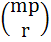Let p be a prime and m be a positive integer. By mathematical induction on m, or otherwise, prove that whenever r is an integer such that p does not divide r, p divides